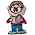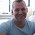## Friday, May 01, 2009

### Flu Like Symptoms

A doctor is called in to see a sick kid. The doctor knows that 90% of the sick kids in the neighborhood have the flu, while the other 10% have the measles. (Assume that's it for diseases right now).

95% of the kids who have the measles develop a rash. Only 8% of the kids with the flu develop a rash.

The doctor, looking at the child, notices a rash. What is the probability the kid has the measles?

1.What? No one even tried? That's unusual. I'll give it another day before I post the answer.

2.taking no of kids as 100
10 kids out of hundred have measles. so prob is 10/100 or 1/10.
out of these 10 kids 95% develop a rash that is 9.5 kids

ans: 9.5/100...

am i correct?

3.The probability of having a rash given you have the measles is 0.95. We'll call this P(R|M).

The probability of having the measles, P(M), is 0.10.

The probability of having a rash given you have the flu, P(R|F) is 0.08.

The probability you have the flu, P(F) is 0.90.

So, the probability of having the measles given you have a rash, P(M|R), can be found using Bayes formula.

P(M|R) = P(R|M) P(M) / (P(R|M) P(M) + P(R|F) P(F))
= .95 × .10 / (.95 × .10 + .08 × .90)
≈ 0.57.

4.Continuing the questionnaire,

Let's say Alvin will catch the flu with probability of 1/10 during any given month. Let's also assume that Alvin can catch the flu only once per month, and that if he has caught the flu, the flu virus will die by the end of the month. What is the probability of the following events?

He catches the flu in September, October and November.

He catches the flu in September and then again in November, but not in October

He catches the flu exactly once in the three months from September through November.

He catches the flu in two or more of the three months from September through November.

we'll see if anyone can answer this.

1.1/1000,
9/1000,
3* (81/1000) = 243/1000
3* (9/1000) + 1/1000 = 7/250

Leave your answer or, if you want to post a question of your own, send me an e-mail. Look in the about section to find my e-mail address. If it's new, I'll post it soon.

Please don't leave spam or 'Awesome blog, come visit mine' messages. I'll delete them soon after.

Enter your Email and join hundreds of others who get their Question of the Day sent right to their mailbox

The Lamplight Manor Puzz 3-D